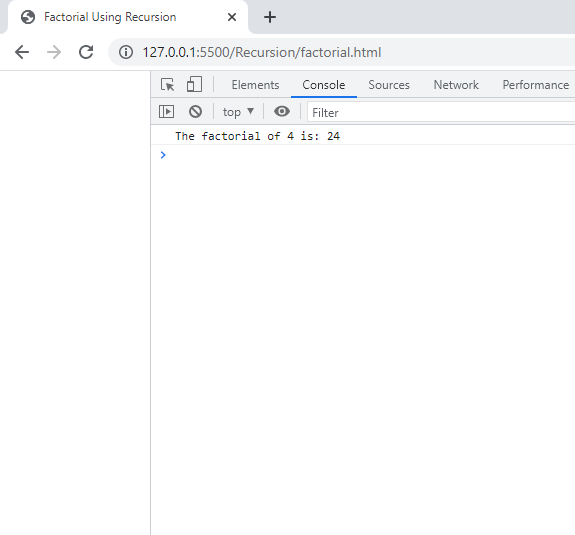# Recursive Function In JavaScript

in STEMGeeks2 years agoImage Source

Recursion is a technique that repetitively divides a task into a smaller tasks also called as subtasks as per specified condition until a solution to simplest subtask is found. It then backtracks with that simplest subtask solution to find the solution of your task. So getting back to point, recursive functions are those functions that call itself. In general, it is a bad practice to use recursion if you don't know the suitable condition to exit from the recursion call. It will crash your program by running out of memory as it will go into infinite loop if condition is not properly defined. By analyzing this way, one can make the way of using recursion more efficient.

You can use recursion to solve many problems relating to Fibonacci sequence, finding factorial of a number, finding greatest common divisor (aka HCF) of a number and so on. Even in the bigger real life project, recursion is used like searching a file system. Here, we will see a simple recursive function that can be used to calculate factorial of a number in JavaScript. This code is very simple and doesn't involve any JavaScript DOM manipulation.

``````<!DOCTYPE html>
<html>
<title>Factorial Using Recursion</title>
<script>
function findFactorial(n)
{
if (n==0)
{
return 1;
}
else if (n==1)
{
return 1;
}
else
{
return n * findFactorial(n-1);
}
}

result_output = findFactorial(4);
console.log("The factorial of 4 is: " + result_output);
</script>
<body>
</body>
</html>
``````

In the above code we specified a condition inside a function that if number is 0 and 1 then it should return 1 as factorial of 0 is 1 and factorial of 1 is also 1. One condition is placed in original if-block and the other one in else-if block. And any other number besides 0 and 1, we use a recursive function. We can see the above code finds factorial of 4. In mathematics, we write it as 4! = 4 * 3!. So, in order to find factorial of 4, we need to have factorial of 3. And in the same way, in order to find the factorial of 3, we need to have factorial of 2. In order to find the factorial of 2, we need to have factorial of 1. At this point, we have solution for factorial of 1 which is 1. So it backtracks to find factorial of 2 then to 3 and then finally to 4. There we have our final solution.

When you open your browser console then you can see the following output: